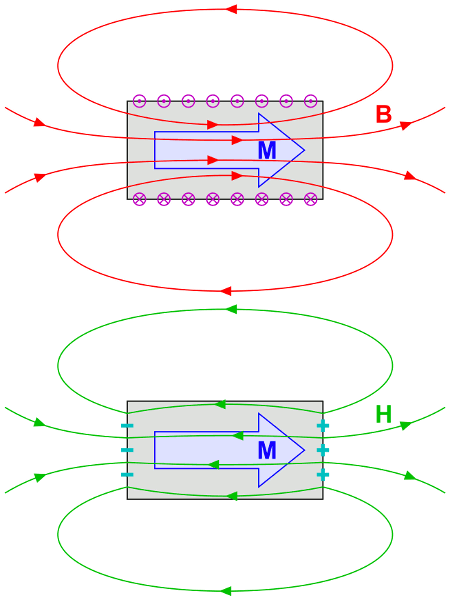# Can you solve this under a minute?A $3C$ charge moving with a velocity of $10m/s$ enters a magnetic field of strength $5 T$ . If its velocity makes and angle of ${ 30 }^{ o }$ with the field , then the Lorentz force experienced by the charge is

×